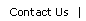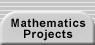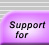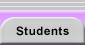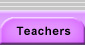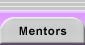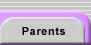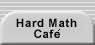# FRACTION EQUATIONS AND OTHER RESEARCH SETTINGS

Research settings differ from research projects in that they do not start with an initial guiding question. Settings begin with one or more mathematical objects and leave it to the researcher to make observations and to define the problem that those objects inspire. You can turn almost any problem into a setting by isolating a single example, by generalizing the problem, or by "neutralizing" the question (e.g., turn "Find the equation for the line with slope 3 passing through the point (1,2)" into "A line goes through the point (1,2). What questions can you ask?"). Here are three settings that involve familiar mathematical objects (fractions, polynomials and integers, and quadrilaterals) and that yield new discoveries and approaches every time a new group works on them. Enjoy!

## FRACTION EQUATIONS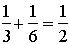• What is this equation an example of?
• Can you find any other equations like it?
• What makes them alike?

## POLYNOMIALS AND FACTORS

xn – 1

xn + 1

x2 + x + n

Pick one of these families of polynomials (or come up with your own) and look at the polynomial expression for different whole number values of n. For each instance of n, look at the values and factors of the expression for whole number values of x.

For example, if we pick xn – 1, we can make a table like this:

 n n = 2 n = 3 n = 4 n = 5 xn + 1 x2 + 1 x3 + 1 x4 + 1 x5 + 1 x Value Factors Value Factors Value Factors Value Factors 1 2 2 2 2 2 2 2 2 2 5 5 9 32 17 17 33 3, 11 3 10 2, 5 28 22, 7 82 2, 41 244 22, 61 4 15 3, 5 65 5, 13 257 257 1025 52, 41 5 24 23, 3 126 2, 32, 7 626 2, 313 3126 2, 3, 521 6 37 37 217 7, 31 1297 1297 7777 7, 11, 101

What patterns can you discover, what questions can you ask, and what explanations can you provide?

See the Quadrilateral Explorations described in the Proof chapter of the teacher handbook.

## TEACHER NOTES

Warning! Please do not read further if you plan to work on these settings yourself. They may ruin your fun.

### Fraction Equations Notes

Investigators take this problem in a number of directions:

• Are there other fractions with a numerator of one (called unit fractions) that can be written as the sum of two other unit fractions?
• Are there other unit fractions that can be written as the sum of any number of distinct unit fractions?
• Which fractions (regardless of the numerator) can be written as the sum of unit fractions?
• Are there other sums where one denominator is the product of the other two?

For those pursuing the first question, are there relationships between your different examples? Can you identify different families of triples (of denominators)? Is the equation 1/10 + 1/15 = 1/6 a member of any of your families?

An internet search for "unit fractions" or "Egyptian fractions" will provide a great deal of information about this setting.

### Polynomials and Factors Notes

Look for patterns in both the rows and columns:

• What factors seem to appear frequently in a given row?
• How do the numbers of factors vary by column?
• What other number types seem to be more or less plentiful in different rows and columns?

What do the factorizations of the polynomials themselves tell us about these apparent patterns?

Thinking about divisibility and modular arithmetic can help. You can represent odds and evens algebraically using 2k and 2k +1. If you see patterns that appear with a different periodicity, you can represent numbers in a similar fashion. For example, if patterns follow a cycle every 6 values, you can explore them using 6k + r (with 0r < 6).

Computer Algebra Systems (CAS) such as the TI-89 and TI-92 calculators, TI Interactive, Maple, or Mathematica are invaluable tools for this exploration. For example, in Matheamtica, the command "FactorInteger[Range^2+Range+1]" will provide the factorization for all x from 1 to 100 for the polynomial x2 + x + 1. Other commands will let you count the number of factors or test for the presence of particular factors for all 100 factorizations at once.

See Factoris at http://wims.unice.fr/wims/wims.cgi?lang=en&+module=tool%2Falgebra%2Ffactor.en for an interactive tool that factors polynomials and integers.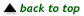Translations of mathematical formulas for web display were created by tex4ht. © Copyright 2003 Education Development Center, Inc. (EDC)